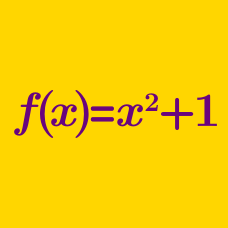Algebra

# Evaluating Functions

If $f(x) = 2x,$ what is the value of $f(-4)?$

If $f(x) = ax,$ what is the value of $a$ satisfying $f(-1) = 3?$

Two functions $f$ and $g$ satisfy the following relationships.

\begin{aligned} f(1)= 2 \\ f(2)= 1 \\ f(3)= 2 \\ f(4)= 5 \\ g(1)= 3 \\ g(2)= 5 \\ g(3)= 7 \\ g(4)= 9 \\ \end{aligned}

What is the value of $(f \circ g)(1)$ ?

Let $\mathbb{R}^+$ be the set of all positive real numbers, and let $f:\mathbb{R}^+ \to \mathbb{R}$ be defined by

$f(x)= \begin{cases} \frac{1}{q} & \text{ if } x= \frac{p}{q}, \\ 0 & \text{ if } x \text{ is an irrational number}, \end{cases}$

where $p$ and $q$ are coprime positive integers. What is the value of $f\left(\frac{12}{13}\right)+f(\pi)+f(0.75)?$

If $f(x) = 2x + a,$ what is the value of $a$ satisfying $f(2) = 2?$

×